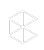Learn from the best and brightest in legal, technology, and beyond! Join us at the 2019 Clio Cloud Conference — San Diego | October 21-22, 2019.

How is Compound Interest Calculated?

Objective:

• How does Clio calculate compound interest?

Environment:

• Clio Web App
• Payment Profiles

n/a

The rate you enter is the Nominal Annual Interest rate—the effective rate will be slightly higher due to the effect of the compounding period.

At the end of each interest period:

New Interest = Current Amount Outstanding * Nominal Annual Rate * (# days in interest period / 365)

New Amount Outstanding = Current Amount Outstanding + New Interest

Example:

30 day interest period, 12% nominal annual rate, \$1000 outstanding

After 30 days, New Interest = 1000 * 0.12 * (30/365) = 9.863

New Amount Outstanding = 1000 + 9.863 = \$1009.86